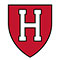Mathematics for Machine Learning

# Mathematics for Machine Learning

clickHere
Updated on Sep 15, 2023 14:36 IST

Mathematics is the backbone of machine learning. Concepts like linear algebra, calculus, and probability theory help you use algorithms, optimize models, and analyze data better.
Don’t think it’s only about memorizing formulas. They will help you grasp the fundamental principles behind building and training intelligent systems.
Mastering these mathematical foundations is crucial to solve complex ML-based real-world problems.

Mathematics and Machine Learning are two words that complement each other, and both always give mere thoughts to all learners. But don’t worry; this blog will help you learn how and what to learn in mathematics to conquer the concepts of Machine Learning.

We will break down key concepts such as Linear Algebra, Probability & Statistics, and Calculus, showing you their relevance to the exciting world of Machine Learning.

Must Check: Mathematics for Data Science

So, let’s get started!!

But before going to what to learn in mathematics, let’s discuss why mathematics is important for machine learning and the proper way to learn it.

## Pre-requisite to learn Mathematics for Machine Learning

Basic Mathematics: To understand the concepts of mathematics for machine learning, you must have completed a high-school mathematics course that includes algebra, trigonometry, probability & statistics, and basic calculus.

Basic Programming Skills: Programming is essential for implementing machine learning algorithms and experimenting with mathematical concepts. You must know at least one programming language, Python or R.

Critical Thinking and Problem-Solving Skills: Mathematics requires logical reasoning and problem-solving skills. You can improve your critical thinking by practicing mathematical problems and puzzles.

## Why is Mathematics Important for Machine Learning?

Mathematics is critically important for machine learning as it is the backbone of machine learning, providing the foundation for algorithms and models. It’s like the secret language that machines use to unlock the mysteries of data.

Let’s explore some other reasons to know the importance of Mathematics in Machine Learning:

• Machine Learning Algorithms are based on mathematical principles. Understanding these algorithms requires comprehension of the underlying mathematical concept.
• Mathematical equations and functions are used to represent the relationship between the input features and output predictions. These are important for model building.
• Machine learning models are optimized to find optimal parameters to maximize a performance metric or minimize a cost function.
• Algorithms like gradient descent require a high understanding of differential calculus.
•  Linear Algebra is central to data manipulation in machine learning. It is typically represented as vectors and matrices, and mathematical operations like matrix multiplication are used to preprocess and transform data.
• Concepts like probability distribution, hypothesis testing, and confidence interval of Statistics are used to analyze data distribution, make inferences about populations, and assess the signification of model predictions.
• Mathematics helps you to understand the trade-off between model complexity and generalization.
• Mathematical metrics, such as accuracy, precision, recall, and F1-score, are used to evaluate the performance of machine learning models.

Till now, you know the prerequisite and the importance of mathematics in Machine Learning.

Until now, you know the prerequisite to learning mathematics for machine learning and why mathematics is important.

Now, the question is:

## How Much Mathematics Require for Machine Learning?

The answer is that you don’t have to be an expert mathematician or statistician to master the concepts of Machine Learning. There are a lot of mathematical libraries that are available to make your work easy. But the sound knowledge of mathematics always gives you an edge to knowing what’s happening behind the scenes.

The mathematical concepts used in Machine Learning are Linear Algebra, Statistics and probability, and Calculus.

So, let’s discuss them one by one:

### Linear Algebra: The Building Block

Have you ever wondered how data is structured and managed with the machine learning system?

This is where Linear Algebra comes into the picture. Linear Algebra is used to represent and manipulate data through matrices and vectors.

To master the topics of linear algebra, start with the topics like vector space, matrices, and eigenvalues.

### Statistics & Probability: Steering Insights

Have you ever thought about how Machine Learning models make predictions or classifications?

Statistics and Probability are used to analyze data and make informed decisions. They enable you to quantify uncertainty and calculate the probability of certain outcomes.

Some common application of statistics and probability in machine learning involves weather forecasting and making sports strategies.

#### Topics You Can Explore in Statistics and Probabilityfor Machine Learning

Now, it’s time to discuss the rockstar of mathematics, i.e., Calculus.

### Calculus: The Force Behind Optimization

Calculus is the branch of mathematics that calculates the instantaneous rate of change, which helps you optimize the model’s performance.

Using calculus, you can find the maximum and minimum to fine-tune your algorithm to achieve peak performance.

To learn calculus concepts, follow the sequence: limit, continuity, differentiation, integration, and multivariate calculus.

clickHere

Vikram has a Postgraduate degree in Applied Mathematics, with a keen interest in Data Science and Machine Learning. He has experience of 2+ years in content creation in Mathematics, Statistics, Data Science, and Mac... Read Full Bio

## Trending Data Science CoursesMachine Learning
Coursera4.6Supervised Machine Learning: Regression and ClassificationSupervised Machine Learning: Regression and Classi...
Coursera5.0Starts today

## Top Picks & New Arrivals